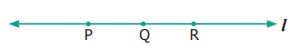Home | | Maths 6th Std | Exercise 4.3

# Exercise 4.3

6th Maths : Term 1 Unit 4 : Geometry : Exercise 4.3 : Text Book Back Exercises Questions with Answers, Solution

Exercise 4.3

1. Observe the diagram and fill in the blanks.(i) ‘A’, ‘O’ and ‘B’ are Collinear points.

(ii) ‘A’, ‘O’ and ‘C’ are Non−collinear points.

(iii) ‘A’, ‘B’ and ‘C’ are non−collinear points.

(iv) O is the point of concurrency.

2. Draw any line and mark any 3 points that are collinear.3. Draw any line and mark any 4 points that are not collinear.4. Draw any 3 lines to have a point of concurrency.5. Draw any 3 lines that are not concurrent. Find the number of points of intersection.Objective Type Questions6. A set of collinear points in the figure are _________.

(a) A, B, C

(b) A, F, C

(c) B, C, D

(d) A, C, D

7. A set of non-collinear points in the figure are _________.

(a) A, F, C

(b) B, F, D

(c) E, F, G

(d) A, D, C

8. A point of concurrency in the figure is _________.

(a) E

(b) F

(c) G

(d) H

Exercise 4.3

1) i) Collinear ii) Non-Collinear iii) Non-Collinear iv) °

2)3)4)5)Objective Type Questions

6) b) AFC

7) d) A, D, C

8) b) F

Tags : Questions with Answers, Solution | Geometry | Term 1 Chapter 4 | 6th Maths , 6th Maths : Term 1 Unit 4 : Geometry
Study Material, Lecturing Notes, Assignment, Reference, Wiki description explanation, brief detail
6th Maths : Term 1 Unit 4 : Geometry : Exercise 4.3 | Questions with Answers, Solution | Geometry | Term 1 Chapter 4 | 6th Maths